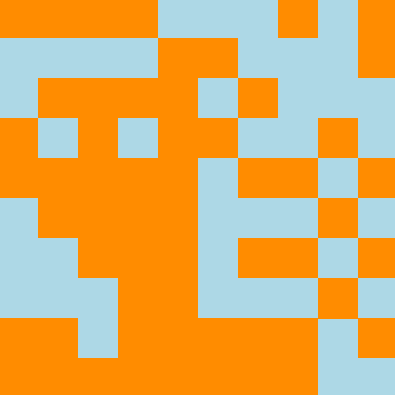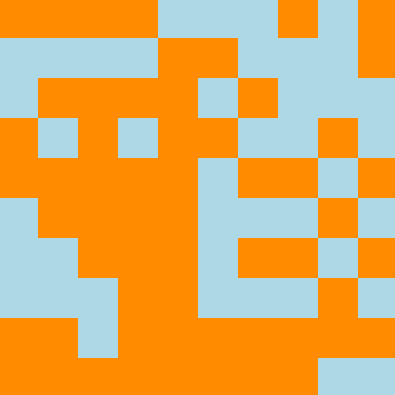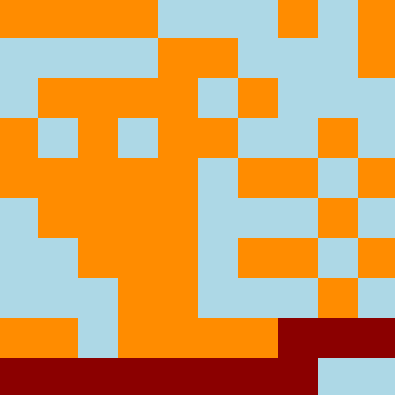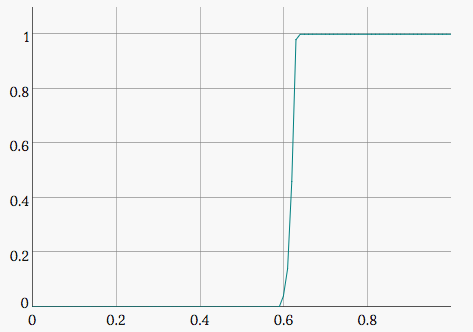# Percolation

From wikipedia: In physics, chemistry and materials science, percolation refers to the movement and filtering of fluids through porous materials. Original problem was described by Broadbent and Hammersley in 1957: Will the water reach the center of the porous rock when it is submerged under water?

Percolation theory studies such models. It can be modelled in two ways.

#### Bond percolation

An edge or a bond between two sites may be open with probability `p` or closed with probability `1 - p`. What is the probability that a path over open bonds exists from one side to another?

#### Site percolation

Another way to model is to say that a site itself is open with probability `p` or closed with probability `1 - p`. What is the probability that a path over open sites exits from one side to another?

Note: in this post connectivity is examied between left and right sides.

Example of site percolation simulation with `p = 0.59` (blue site are closed, orange are open):As you can see, there is no path from left to right over open (orange) sites. But if one site in lower right becomes openthen site percolation is observed:#### Critical percolation threshold

In case of infinite network, for any given probability `p` the probability that an infinite open cluster exists is either zero or one meaning that there must be critical `pc` below which the probability of an infinite open cluster is zero and above is one.

Example of site percolation probability given probability `p` to enable a single site for n = 400://: # ( T = new Dygraph(document.getElementById(“prob_plot”), ) //: # ( “prob.txt”, ) //: # ( { ) //: # ( fillAlpha: 0.50, ) //: # ( axes: { ) //: # ( x: { ) //: # ( axisLabelFormatter: function(x) { ) //: # ( var shift = Math.pow(10, 5) ) //: # ( return Math.round(x * shift) / shift ) //: # ( } ) //: # ( } ) //: # ( } ) //: # ( }); ) //: # ( )

As you can see the critical probability `pc` for 2D site percolation is between `0.59` and `0.60`.

You can play with site percolation here.